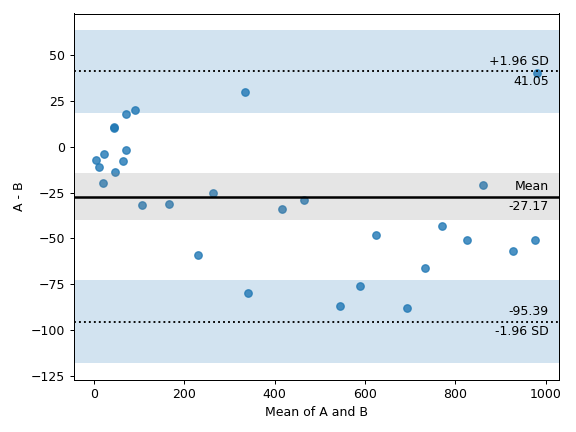# pingouin.plot_blandaltman

pingouin.plot_blandaltman(x, y, agreement=1.96, confidence=0.95, figsize=5, 4, dpi=100, ax=None)[source]

Generate a Bland-Altman plot to compare two sets of measurements.

Parameters
x, ynp.array or list

First and second measurements.

agreementfloat

Multiple of the standard deviation to plot limit of agreement bounds. The defaults is 1.96.

confidencefloat

If not None, plot the specified percentage confidence interval on the mean and limits of agreement.

figsizetuple

Figsize in inches

dpiint

Resolution of the figure in dots per inches.

axmatplotlib axes

Axis on which to draw the plot

Returns
axMatplotlib Axes instance

Returns the Axes object with the plot for further tweaking.

Notes

Bland-Altman plots  are extensively used to evaluate the agreement among two different instruments or two measurements techniques. They allow identification of any systematic difference between the measurements (i.e., fixed bias) or possible outliers.

The mean difference is the estimated bias, and the SD of the differences measures the random fluctuations around this mean. If the mean value of the difference differs significantly from 0 on the basis of a 1-sample t-test, this indicates the presence of fixed bias. If there is a consistent bias, it can be adjusted for by subtracting the mean difference from the new method.

It is common to compute 95% limits of agreement for each comparison (average difference ± 1.96 standard deviation of the difference), which tells us how far apart measurements by 2 methods were more likely to be for most individuals. If the differences within mean ± 1.96 SD are not clinically important, the two methods may be used interchangeably. The 95% limits of agreement can be unreliable estimates of the population parameters especially for small sample sizes so, when comparing methods or assessing repeatability, it is important to calculate confidence intervals for 95% limits of agreement.

The code is an adaptation of the PyCompare package. The present implementation is a simplified version; please refer to the original package for more advanced functionalities.

References

1

Bland, J. M., & Altman, D. (1986). Statistical methods for assessing agreement between two methods of clinical measurement. The lancet, 327(8476), 307-310.

Examples

Bland-Altman plot

>>> import numpy as np
>>> import pingouin as pg
>>> np.random.seed(123)
>>> mean, cov = [10, 11], [[1, 0.8], [0.8, 1]]
>>> x, y = np.random.multivariate_normal(mean, cov, 30).T
>>> ax = pg.plot_blandaltman(x, y)